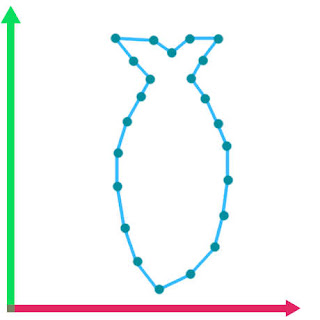### How To Animate A Fish Swimming With Shaders

We are going to make swimming animation by using only shader code.
By the time we are done, it's going to look like this.Pretty Damn Cool...
You will probably need the fish model used in this tutorial, that can be found HERE. Can use your own model but the shader code might have to be modified accordingly because of the orientation of the model that you might be using ( issues with whether the X axis & Z axis is flipped ).
The shader used way out performs a similar scene with skeletal animations applied on the fish models.
On a previous benchmark I did comparing the shader animation with the skeletal animation there was a difference of 28 FPS( on average ) with 50 fish.
The shader we are going to make is really powerful and flexible and don't think that it's limited to making fishes swim😀.Y-Z Axis
So this mesh oriented like this when imported into unity and this is important to understand because this means that the model's vertices have to be moved along the X-axis.
Also because this is done with the vertex shader, you have to use to use a model that has enough vertex density otherwise the effect falls flat ( might have to tessellate in that case ).
Coming to how the fish swims,
The head of the fish doesn't really move that much and as we go along the length of the body the more the movement.
So the basic motion can be emulated with a Sin function but how do we make the motion near the head less than at the tail end?
Well... we use some kind of gradient that makes it so that it prevents motion near the head and promotes motion at the tail.This gradient will ensure proper look to the fish body motion.
But there is one more key ingredient that makes the entire thing more believable... and that is side to side motion ( I'm calling it stride in the shader ) of the fish as it's moving through the water.
If we combine all of this then we are golden.
We will first look at the properties of the shader.
``````Properties
{
_MainTex ("Texture", 2D) = "white" {}
_WaveSpeed("Wave Speed", Range(0.0,100.0)) = 3.0
_WaveHeight("Wave Height", Range(0.0,30.0)) = 5.0
_WaveDensity("Wave Density", Range(0.0001,1.0)) = 0.007
_Yoffset("Y Offset",Float) = 0.0
_Threshold("Threshold",Range(0,30)) = 3
_StrideSpeed("Stride Speed",Range(0.0,10.0)) = 2.0
_StrideStrength("Stride Strength", Range(0.0,20.0)) = 3.0
_MoveOffset("Move Offset",Float) = 0.0
}
``````
We will see what, why & when these properties are used.
What They Are:
``````sampler2D _MainTex;
float4 _MainTex_ST;
half _WaveSpeed;
half _WaveHeight;
half _WaveDensity;
half _Yoffset;
int _Threshold;
half _StrideSpeed;
half _StrideStrength;
half _MoveOffset;
``````
Let's Look At The Structs
``````struct appdata
{
float4 vertex : POSITION;
float2 uv : TEXCOORD0;
};
struct v2f
{
float2 uv : TEXCOORD0;
UNITY_FOG_COORDS(1) // Keep Or Remove This On Basis Of Fog Requirement
float4 vertex : SV_POSITION;
};
``````
Now we will see why & when these come in to play, which so happens to be the vertex shader.
``````v2f vert (appdata v)
{
v2f o;
half sinUse = sin(-_Time.y * _WaveSpeed + _MoveOffset + v.vertex.y * _WaveDensity);
half yValue = v.vertex.y - _Yoffset;
half yDirScaling = clamp(pow(yValue * _EffectRadius,_Threshold),0.0,1.0);
v.vertex.x = v.vertex.x + sinUse * _WaveHeight* yDirScaling;
v.vertex.x = v.vertex.x + sin(-_Time.y * _StrideSpeed + _MoveOffset) * _StrideStrength;
o.vertex = UnityObjectToClipPos(v.vertex);
o.uv = TRANSFORM_TEX(v.uv, _MainTex);
UNITY_TRANSFER_FOG(o,o.vertex);
return o;
}``````
Time to break it down.
``half sinUse = sin(-_Time.y * _WaveSpeed + _MoveOffset + v.vertex.y * _WaveDensity);``
-Time.y * _WaveSpeed :- basically determines how fast the Sin waves are made and -ve sign means that waves look as if it's moving in +ve Y axis in model space.
v.vertex.y * _WaveDensity :- determines how much frequency the Sin waves have.
_MoveOffset :- this adds variation between each fish, otherwise all fish would move the same.
``half yValue = v.vertex.y - _Yoffset;``
Determines the model space Y position from which we perform calculations.
``half yDirScaling = clamp(pow(yValue * _EffectRadius,_Threshold),0.0,1.0);``
yValue * _EffectRadius:-The area which is going to be affected by the Sin function.
``pow(yValue * _EffectRadius,_Threshold)``
The returned value is how smooth the transition is from the area affected by the Sin function and the area that is not and this is determined by the _Threshold value.
``clamp( pow( yValue * _EffectRadius,_Threshold ),0.0,1.0)``
Makes sure that the values are within 0.0 to 1.0 range.
``v.vertex.x = v.vertex.x + sinUse * _WaveHeight * yDirScaling;``
Now the X - Coordinate of the vertex will be moved : _WaveHeight * yDirScaling will determine what the overall displacement is, then the sinUse value will multiply the calculated Sin function value on to this.
``v.vertex.x = v.vertex.x + sin(-_Time.y * _StrideSpeed + _MoveOffset) * _StrideStrength;``
The vertex is again displaced along the X-axis but this time it doesn't not change with the Y-axis so...all the vertices move equally in the X-axis.
``sin(-_Time.y * _StrideSpeed + _MoveOffset)``
The _MoveOffset value plays a role again here in making it look unique.
``o.vertex = UnityObjectToClipPos(v.vertex);``
Now provides the output variable the Clip position on screen from that vertex data.
Since we can't give each fish a unique _MoveOffset value from editor as it will override that material value for all the fish. So a small C# script can be used.
``````using UnityEngine;

public class FishMotion : MonoBehaviour
{
private Material fishMaterial;
void Start ()
{
fishMaterial = GetComponent().material;
fishMaterial.SetFloat("_MoveOffset", Random.Range(0.0f, 3.14f));
}
}
``````
Just attach this as component to all the fish ( or whatever ) in the scene.
The entire shader source code is available HERE.
The Unity package available HERE.
If you like programming shaders make sure you check these out : Shader Tutorials
Support Bitshift Programmer by leaving a like on Bitshift Programmer Facebook Page and be updated as soon as there is a new blog post.